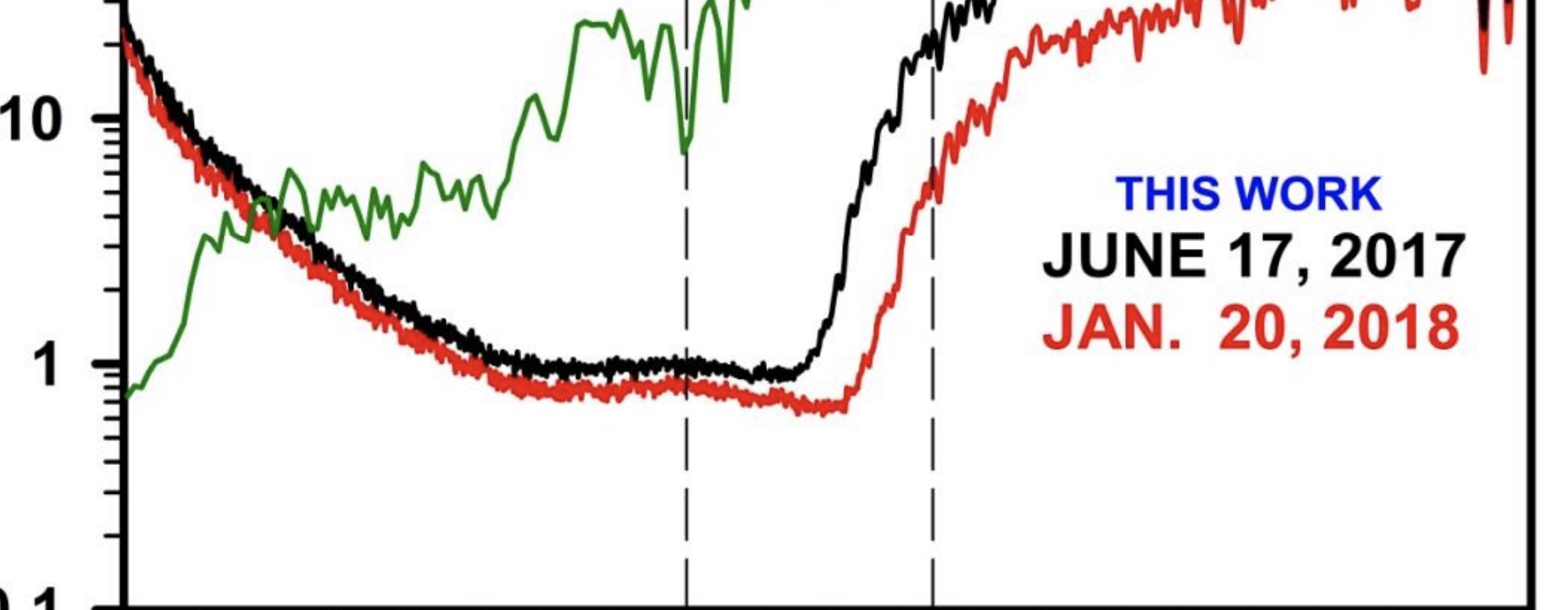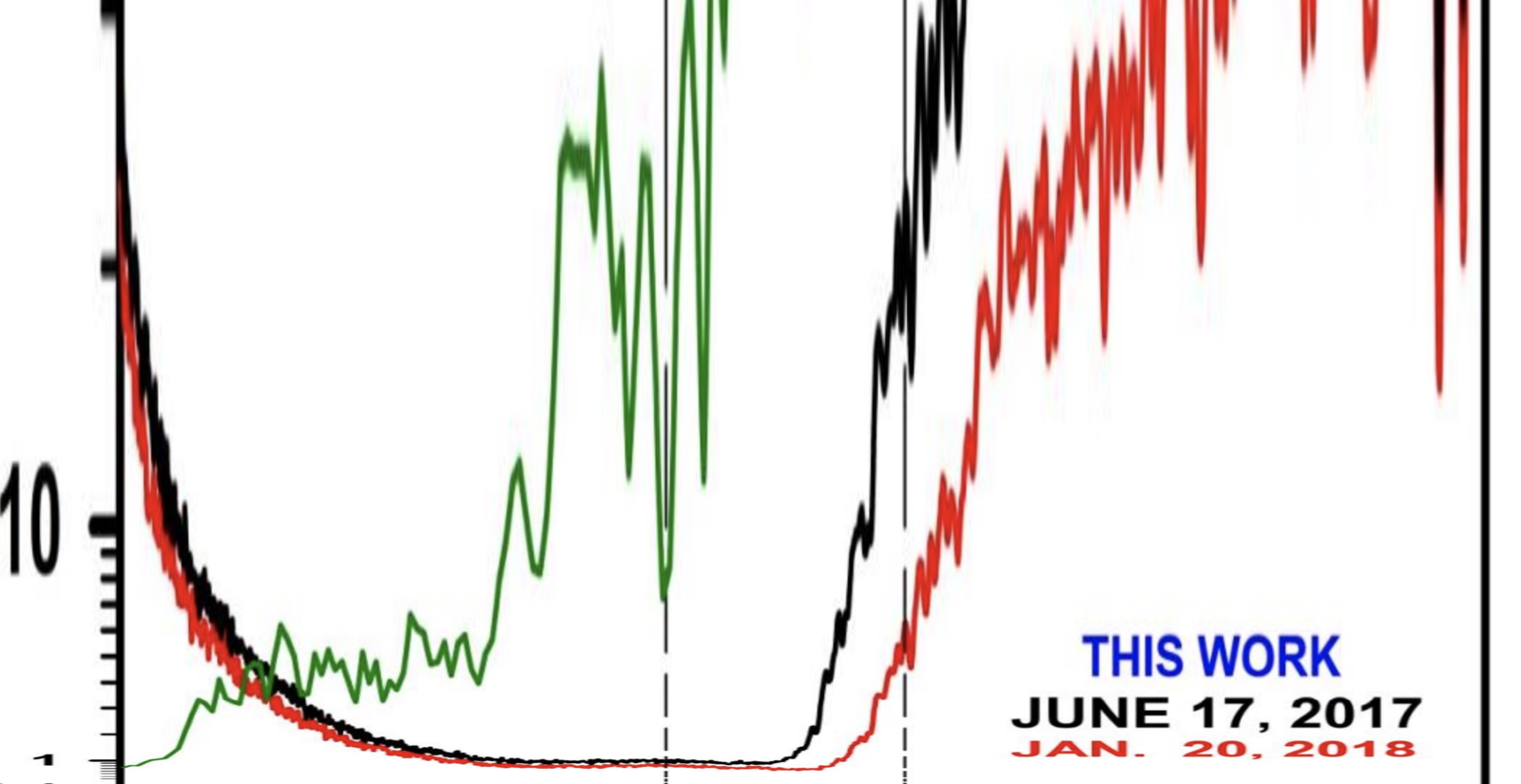# Convert Graph Image from Log Scale to Linear Scale

#### Mick West

Staff memberSometimes graphs are presented with a logarithmic vertical scale. This is useful for seeing the detail in the smaller range, but can give a misleading impression about how much a value is changing.

I wrote a no-frills tool to convert such images. It take as input a URL of a cropped portion of a graph and the bottom and top values of the y axis. For example.

https://www.metabunk.org/delog/?img...org/delog/herdon-v-NASA-0.1-30.jpg&a=0.1&b=30Notice there how the red and black lines still drop down to just below 1, but now we can see on the larger scheme of things it's pretty much zero. The log scale made it look more significant. Also we can see the peak to the left (an artifact of stray light being magnified in software to account for decreasing sensitivity) is more polynomial than linear.

The code is relatively short, although the math was slightly headscratchy. I might extend it to drag-and-drop if needed. But this is an infrequently used tool.
Code:
``````// each line in the image of height h is k * previous line larger, where k is something like 1.02343
// we have start * k^h = end
// so k^h = end/start
// ln(k)*h = ln(end/start)
// k = exp(ln(end/start))/h

var h = img.height;
var k = Math.exp(Math.log(end / start) / (h))
console.log("n=" + n + ", k = " + k)

var n = canvas.height;  // n = number of lines

var log_pos = start;

// for each line in the canvas
for (var line = 0; line < n; ++line) {

// v is value that goes 0 to 1 down the canvas
var v = line / canvas.height;

// convert that to a y value in the graph
var y = start + (1 - v) * (end - start);

// now convert that to a line number g in the log graph
// we know y = start*k^g
// so ln(y) = ln(start)+ln(k)*g
// g = (ln(y) - ln(start))/ln(k)

//				var srcY = img.height - (Math.log(y) - Math.log(start))/Math.log(k)
// simplifies slightly as
var srcY = h - (Math.log(y) - Math.log(start)) / (Math.log(end / start) / (h))

// clamp srcY to be inside the image
srcY = Math.max(0, Math.min(img.height - 1, srcY));

// draw a single line from the src to the canvas
ctx.drawImage(
img,
0, srcY, img.width, 1,
0, line, canvas.width, 1);
}``````

Feel free to reuse this code